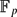Mathematical Proceedings of the Cambridge Philosophical Society ( IF 0.741 ) Pub Date : 2018-10-24 , DOI: 10.1017/s0305004118000798
G. CONANT; A. PILLAY; C. TERRY

We prove that, given ε > 0 and k ≥ 1, there is an integer n such that the following holds. Suppose G is a finite group and AG is k-stable. Then there is a normal subgroup HG of index at most n, and a set YG, which is a union of cosets of H, such that |AY| ≤ε|H|. It follows that, for any coset C of H, either |CA|≤ ε|H| or |C \ A| ≤ ε |H|. This qualitatively generalises recent work of Terry and Wolf on vector spaces over$\mathbb{F}_p$ .

down
wechat
bug